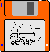### JPL's Wireless Communication Reference Website

#### Chapter: Wireless Channels Section: Multipath Fading# Scatter Function

Multipath fading and user mobility lead to a time and frequency dependent channel. The Transfer function of a particular sample channel does not necessarily provide enough details about the stochastic behavior of the radio channel. Such stochastic properties are captured in the scatter function. The scatter function combines information about
The scatter function provides a statistical model for the channel.

 Figure: the basic idea behind the scatter function is that it plots the expected power per Doppler shift and per excess delay bin. Sometimes, angle of incidence (bearing) is plotted in stead of the Doppler shift.Each path can be described by its

• Angle of arrival or Doppler shift
• Excess delay

Thus we can plot the received energy in a two dimensional plane, with Doppler shift on one horizontal axis and delay on the other horizontal axis.

 Environment Delay Spread Angle spread Max. Doppler shift at 1800 MHz Macrocellular: Rural flat 0.5 ms 1 degree 200 Hz Macrocellular: Urban 5 ms 20 degrees 120 Hz Macrocellular: Hilly 20 ms 30 degrees 200 Hz Microcellular: Factory, Mall 0.3 ms 120 degrees 10 Hz Microcellular: Indoors, Office 0.1 ms 360 degrees 2..6 Hz Table Source: A.J. Paulray and C.B. Papadias, "Space-Time Processing for Wireless Communications", Signal Processing Magazine, November 1997, pp. 49-83.Audio commentary: Peter M. Grant, Distinguished IEEE Lecturer 1997, discusses the table parameters (MPEG audio). See also: Full talk, MPEG plug-in on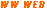## A Practical Example from GermanyFigure: measured scatter plot for DCS 1800 MHz system. Doppler spread = 60.3 Hz; Coherence time = 5.9 msec. Delay Spread = 1.2 msec; coherence BW = 1.3 MHz Source: Research group of Prof. Paul Walter Baier, U. of Kaiserslautern, Germany.Figure: Doppler spread corresponding to above scatter plot. Note that the Doppler spread is the projection of the scatter plot on the Doppler frequency axis.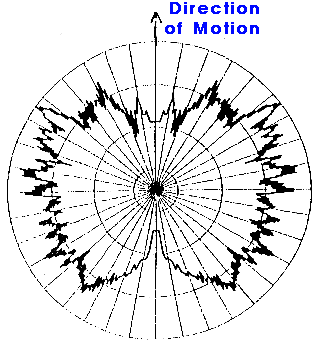Figure: Distribution of angle of arrival corresponding to above scatter plot.Figure: Delay spread profile corresponding to above scatter plot. Note that the Doppler spread is the projection of the scatter plot on the time delay axis.

## An example from EdinburghReceived power (according to color) versus time of arrival (horizontal axis) and angle of incidence (vertical axis). Source credit: Nortel.Audio commentary: Peter M. Grant, Distinguished IEEE Lecturer 1997. MPEG audio: MP2 ** Power versus delay and angle MP2 Measurement artifacts in delay-angle map See also: Full talk, MPEG plug-in on## An indoor example from ZurichA realization of the local Power Delay-Direction Profile (PDDP). Carrier frequency 5.2 GHz, MT velocity 1 m/s, delay spread TRMS = 50 ns. Such a situation is typical for a small room environment. See the PDDP evaluation by Peter E. Leuthold and Pascal Truffer.

## Theoretical Example

Let's consider a
• U-shaped Doppler spectrum, as it occurs with uniformly distributed angles of arrival of reflected waves. The maximum shift is fm.
• an exponential delay spread with mean Trms
Moreover, we assume that the delay spread and Doppler spread are separable. Then the amount of (scatter power) per frequency and time bin can be expressed as
```
Plocal-mean      1               1           t
p(f,t) = ------- --------------------  ---- exp(- -----)
4 p  fm            (f-fc)2     Trms        Trms
sqrt( 1 - -------)
fm2
```
The integral over p(f,t) gives to total received local mean power Plocal-mean.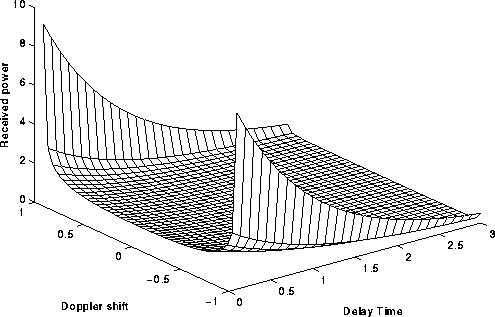Figure: Scatter function. Received power per unit of frequency shift and per unit of excess time delay.
Frequency shift normalized to the maximum Doppler shift.
Delay time normalized to the delay spread.Figure: Scatter function projected to frequency axis. This gives the Doppler spread. Received power per unit of frequency shift.
Frequency shift normalized to the maximum Doppler shift.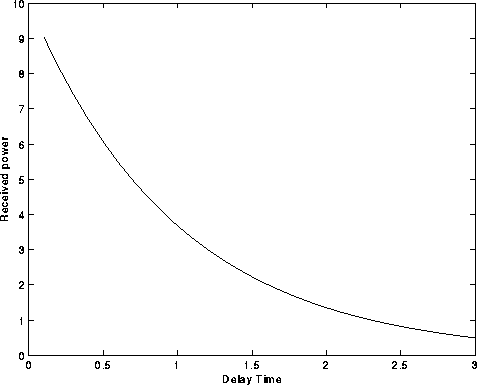Figure: Scatter function project to delay time axis: This gives the delay profile. Received power per unit of excess time delay.
Delay time normalized to the delay spread.

Channel simulations based on this theoretical model have been contributed by Ralph Haas.